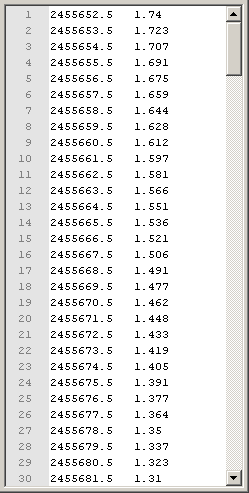Let’s have a look at this variable star called JC4. It has been measured 3 times, on 01-September-2011, 14 and 15-November-2011.

To load the magnitude data in Python one can use the function cdr.load_magdata():

```from aspylib import cdr

#------ input files --------------------------------
Folder = u"C:\\Mesures\\"
Filelist = ["JC4_01Sept2011.txt", "JC4_14Nov2011.txt", "JC4_15Nov2011.txt"]
Filelist = [Folder + x for x in Filelist]

```

The function returns a list with 3 elements, called here “alldata”. Each element in the list is a Numpy array with 3 columns:

• first column contains the Julian dates, shifted at mid-exposure
• second column contains the differential magnitudes for the observed object
• last column contains an estimate of the magnitude noise for each measurement

So the function cdr.load_magdata() not only reads the data from the text files, it also makes several things:

• it shifts the Julian dates to mid-exposure, using the header “POS -1”
• it calculates the differential magnitudes by subtracting the magnitudes of the reference stars
• it calculates a noise estimate

## Time correction for asteroids¶

When measuring asteroid lightcurves, the observation dates need to be corrected from the delay due to light propagation between asteroid and Earth. If one measures lightcurves during several weeks, the correction can amount to several tens of minutes so it is essential to make it.

To make the correction, one needs to prepare a file that contains the variations of the distance Earth-asteroid over a larger period. The information can be copy-pasted directly from the Minor Planet Center ephemeris service.

The file must have the following format:

• two columns, separated with a tabulation
• first column is Julian date, second column is distance Earth-asteroid in AU (Astronomical Units)Then we save the file in the same folder and call it, say, “dist.txt”. The time correction is simply made with:

```from aspylib import cdr

#------ input files --------------------------------
Folder = u"C:\\Mesures\\"
Filelist = ["asteroid_1.txt", "asteroid_2.txt", "asteroid_3.txt"]
Filelist = [Folder + x for x in Filelist]

#------ time correction ----------------------------
alldata = cdr.time_correction(alldata, 1, Folder+'dist.txt')
```

## Time correction for variable stars¶

For variable stars, the time correction is different. We need to shift the Julian dates to the center of the solar system, to remove the changes of distance Earth to star, that is due to the yearly Earth revolution around the Sun. This correction can amount at maximum to about 16 min. It is described here.

To do it we only need the (alpha, delta) coordinates of the star. The time correction is simply made with:

```from aspylib import cdr, astro

#------ input files --------------------------------
Folder = u"C:\\Mesures\\"
Filelist = ["JC4_01Sept2011.txt", "JC4_14Nov2011.txt", "JC4_15Nov2011.txt"]
Filelist = [Folder + x for x in Filelist]

#------ time correction ----------------------------
alpha = astro.hours_to_decdegrees('03:09:39.67')
delta = astro.degrees_to_decdegrees('+24:29:26.9')
alldata = cdr.time_correction(alldata, 2, [alpha,delta])
```